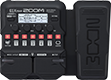# n,chj

Discussion in 'Zoom G1/G1X Four' started by Enrico Seguenti, Jul 22, 2022.

1. n,chjDevice: Zoom G1X Four
Firmware: 2.00

Name on device: AFJA
Optimized for: Phones/Speaker

Effects chain:...And Justice for All rhythm tone

Effect: "ZNR" (Dynamics), active - "yes"
"Detect" = GTRIN
"Depth" = 100
"Threshold" = 35
"Decay" = 0

Effect: "TS Drive" (Overdrive / Distortion), active - "yes"
"Gain" = 0
"Boost" = On
"Tone" = 30
"Volume" = 100

Effect: "DZ DRV" (Amp simulator), active - "yes"
"Bass" = 18
"Middle" = 10
"Treble" = 70
"Presence" = 40
"Gain" = 60
"Volume" = 80
"Deep" = 0
"MidCut" = 50

Effect: "MS4x12GB" (Cabinet), active - "yes"
"MIC" = ON
"D57: D421" = 100
"Hi" = 50
"Lo" = 50

Effect: "Gt GEQ 7" (Filter), active - "yes"
"100" = 4.5
"200" = -4.0
"400" = -4.5
"800" = -12.0
"1.6k" = -7.5
"3.2k" = 2.5
"6.4k" = 11.0
"VOL" = 50

Patch Volume: 50